Documentation

design

Design prototype antenna or arrays for resonance at specified frequency

Description

example

hant = design(antenna,frequency) designs any antenna object from the antenna library to resonate at the specified frequency.

harray = design(array,frequency) designs an array of dipoles for operation at a specified frequency. The elements are separated by half-wavelength.

example

harray = design(array,frequency,elements) designs an array of elements for operation at a specified frequency. The elements are separated by half-wavelength, if possible. If you cannot achieve half-wavelength spacing, the element size is used to calculate inter-element separation and the elements are evenly distributed on a sphere radius proportional to the largest element in element.

harray = design(conformalarray,frequency) designs a conformal array of dipole and bowtie elements at the specified frequency. The elements are placed in the locations specified by default conformalArray object. If the required element positions cannot be achieved due to intersection of elements, the element size is used to compute the inter element spacing and the elements are evenly distributed on a sphere of radius proportional to the largest element in the property Elements.

harray = design(conformalarray,frequency,elements) designs a conformal array of specified elements at the specified frequency.

harray = design(infinitearray,frequency) designs an infinite array with a reflector element at the specified frequency.

harray = design(infinitearray,frequency,elements) designs an infinite array of specified elements at the specified frequency.

Examples

collapse all

Design a prototype microstrip patch antenna that resonates at a frequency of 1 GHz.

p = design(patchMicrostrip,1e9)
p =
patchMicrostrip with properties:

Length: 0.1439
Width: 0.1874
Height: 0.0030
Substrate: [1x1 dielectric]
GroundPlaneLength: 0.2998
GroundPlaneWidth: 0.2998
PatchCenterOffset: [0 0]
FeedOffset: [0.0303 0]
Tilt: 0
TiltAxis: [1 0 0]

show(p)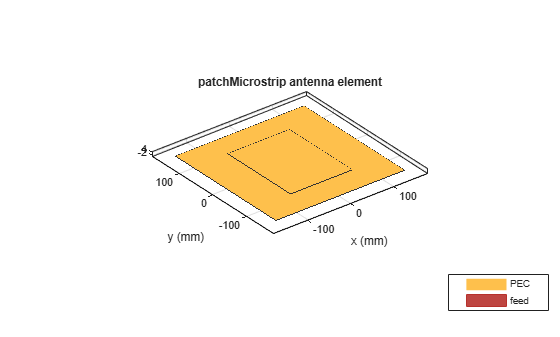Calculate the impedance of the above antenna at the same frequency.

Z = impedance(p,1e9)
Z = 55.8475 - 0.8183i

Design a rectangular array of reflector backed rounded bowtie antennas to operate at 500 MHz.

b = bowtieRounded('Tilt',90,'TiltAxis',[0 1 0]);
r = reflector('Exciter',b);
ra = design(rectangularArray,500e6,r);
show(ra)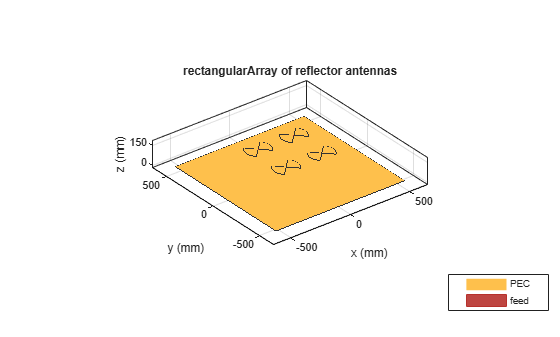Plot the radiation pattern of the rectangular array at 500 MHz.

pattern(ra,500e6)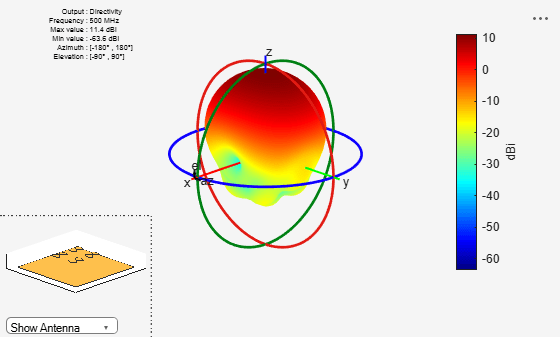Create a default conformal array.

confarraydef = conformalArray
confarraydef =
conformalArray with properties:

Element: {[1×1 dipole]  [1×1 bowtieTriangular]}
ElementPosition: [2×3 double]
Reference: 'feed'
AmplitudeTaper: 1
PhaseShift: 0
Tilt: 0
TiltAxis: [1 0 0]

Add a folded dipole antenna to the conformal array at position [1,1,1].

confarraydef.ElementPosition(3,:) = [1 1 1];
confarraydef.Element{3} = dipoleFolded;
show(confarraydef)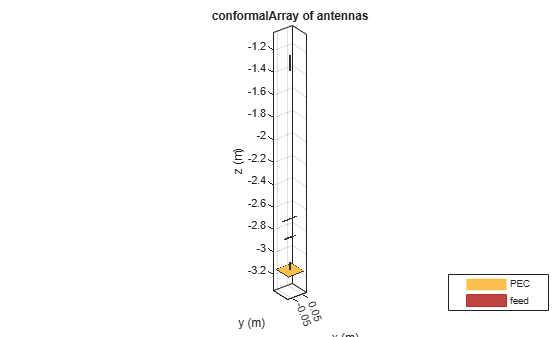Design the above array for a frequency of 1 GHz.

desC = design(confarraydef,1e9)
desC =
conformalArray with properties:

Element: {[1×1 dipole]  [1×1 bowtieTriangular]}
ElementPosition: [2×3 double]
Reference: 'feed'
AmplitudeTaper: 1
PhaseShift: 0
Tilt: 0
TiltAxis: [1 0 0]

desC.ElementPosition
ans = 2×3

0         0         0
0         0    0.1500

show(desC)Create an infinite array.

infarrayV1 = infiniteArray
infarrayV1 =
infiniteArray with properties:

Element: [1×1 reflector]
ScanAzimuth: 0
ScanElevation: 90

show(infarrayV1)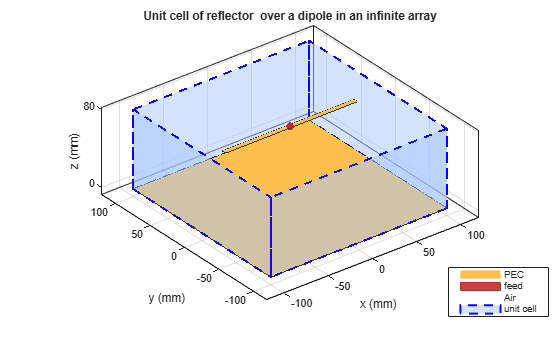Design the above array using a monopole antenna and at 1 GHz frequency.

infarrayV2 = design(infarrayV1,1e9,monopole)
infarrayV2 =
infiniteArray with properties:

Element: [1×1 monopole]
ScanAzimuth: 0
ScanElevation: 90

show(infarrayV2)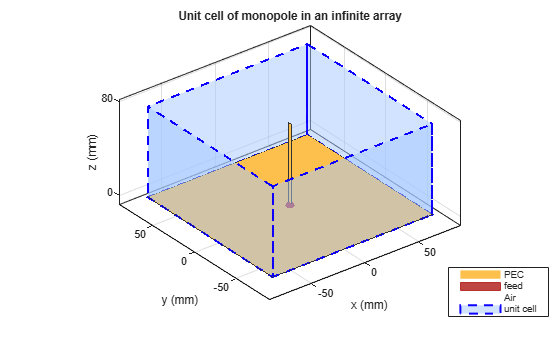Input Arguments

collapse all

Antenna object from antenna library, specified as a scalar handle.

Example: dipole

Array object from antenna library, specified as a linearArray, rectangularArray, or circularArray object.

Example: r = reflector;ra = design(rectangularArray,500e6,r); Designs a rectangular array of reflectors operating at a frequency of 500 MHz.

Conformal array object, specified as a conformalArray object.

You can position elements in a conformal array in three ways:

• Case 1: Points lie on a line.

• Case 2: Points lie on a plane.

• Case 3: Points lie in 3-D space.

Example: c = conformalArray;ca = design(c,50e6,{dipole,dipoleFolded, dipoleJ, bowtieTriangular,dipole,dipole,dipole,dipole,dipole}); Designs a conformal array of specified elements operating at a frequency of 50 MHz.

Infinite array object, specified as a infiniteArray object.

Example: i = infiniteArray;ia = design(1,1e9,monopole); Designs an infinite array with a monopole antenna element operating at a frequency of 1 GHz.

Resonant frequency of the antenna, specified as a real positive scalar.

Example: 55e6

Data Types: double

Antenna object from the antenna library used in the array, specified as a single antenna element or a cell array in conformal array. For more information on element positions for conformal array, see conformalarray.

Example: r = reflector;ra = design(rectangularArray,500e6,r); Designs a rectangular array of reflectors operating at a frequency of 500 MHz.

Example: c = conformalArray;ca = design(c,50e6,{dipole,dipoleFolded, dipoleJ, bowtieTriangular,dipole,dipole,dipole,dipole,dipole}); Designs a conformal array of specified elements operating at a frequency of 50 MHz.

Output Arguments

collapse all

Antenna object operating at the specified reference frequency, returned as an antenna object.

Array object operating at the specified reference frequency and specified elements, returned as an array object.